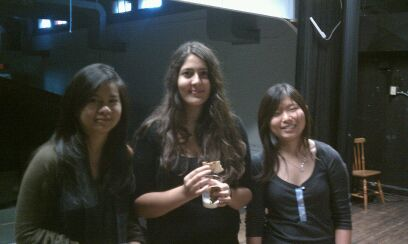"CCS is a unique program with a challenging curriculum. CCS Math promotes group work, not typically emphasized in high school; this skill is essential in the field of research in mathematics. CCS math also gives students a chance to have close relationships with professors." Crystal, Hripsime, and Jane CCS Mathematics Student

# Master Program

A Bachelor’s degree in Mathematics and a Master’s program can be completed by CCS math students in four to five years. The requirements to complete the master’s program are the following:

• The applicant must fulfill the scholarship requirements for graduate study.
• All candidates must complete 42 units with the grade of B or better in each course, 24 units of which must be in graduate course sequences approved and offered by the Department of Mathematics. The remaining 18 units may be in upper-division or graduate-level courses in mathematics or, with the approval of the graduate committee, outside of mathematics, with a limit of 9 units outside the department. The sequences available in the Mathematics Department are the following:
• Real Analysis: Sequence of three courses on Measure Theory and integration. Point set topology. Principles of functional analysis. Lp spaces. The Riesz representation theorem. Topics in real and functional analysis.
• Applied Mathematics. This is a sequence of four courses on the following topics:
• Matrix Analysis and Computation: Graduate level matrix theory with introduction to matrix computations. SVDs, pseudoinverses, variational characterization of eigenvalues, perturbation theory, direct and iterative methods for matrix computations.
• Numerical simulation: Linear multistep methods and Runge-Kutta methods for ordinary differential equations: stability, order and convergence. Stiffness. Differential algebraic equations. Numerical solution of boundary value problems.
• Numerical Solution of Partial Differential Equations - Finite Difference Methods: Finite difference methods for hyperbolic, parabolic and elliptic PDEs, with application to problems in science and engineering. Convergence, consistency, order and stability of finite difference methods. Dissipation and dispersion. Finite volume methods. Software design and adaptativity.
• Numerical Solution of Partial Differential Equations - Finite Element Methods: Weighted residual and finite element methods for the solution of hyperbolic, parabolic and elliptic partial differential equations, with application to problems in science and engineering. Error estimates. Standard and discontinuous Galerkin methods.
• Modern Algebra: Set of three courses on Group theory, ring and module theory, field theory, Galois theory, other topics.
• Topology: A sequence of three course on the following topics:
• Foundations of topology: Metric spaces, topological spaces, continuity, Hausdorff condition, compactness, connectedness, product spaces, quotient spaces. Other topics as time allows.
• Homotopy theory: Homotopy groups, exact sequences, fiber spaces, covering spaces, van Kampen Theorem.
• Differential topology: Topological manifolds, differential manifolds, transversality, tangent bundles, Borsuk-Ulam theorem, orientation and intersection number, Lefchetz fixed point theorem, vector fields.
• Introduction to differential geometry and Riemannian geometry: A sequence of three courses. Topics include geometry of surfaces, manifolds, differential forms, Lie groups, Riemannian manifolds, Levi-Civita connection and curvature, curvature and topology, Hodge theory. Additional topics such as bundles and characteristic classes, spin structures and Dirac operator, comparison theorems in Riemannian geometry.

• Thesis: Students must prepare an acceptable thesis under the supervision of a faculty member and do an oral defense of it before a faculty committee. The 24 graduate units in mathematics must include at least one full-year course sequence that satisfies one of the area requirements.

An example of a Master Thesis defended by a CCS math student recently is:

"The asymptotic cone of the Hilbert metric of a square" by Matt Adam.